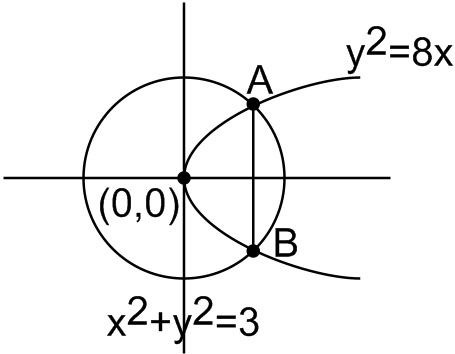#### Let L1 be the length of the common chord of the curves x2 + y2 = 9 and y2 = 8x, and  L2 be the length of the latus rectum of     y2 = 8x, then : Option 1)Option 2)Option 3)Option 4)As we learnt in

Length of the latus rectum -- wherein

For the parabola.For points of intersection ofandSo length oflegnth of Latus Rectum.

Clearly,Option 1)This option is incorrect.

Option 2)This option is incorrect.

Option 3)This option is correct.

Option 4)This option is incorrect.

#### prateek